Performance of Multi-armed Bandit Algorithms

### Basic Setting

The multi-armed bandit problem is a classic reinforcement learning problem which indicated the dilemma between the exploitation and exploration.
We consider a time-slotted bandit system ($t = 0,1,2,\dots$) with three arms. We denote the arm set as $\{1,2,3\}$. Pulling each arm $j$ ($j\in\{1,2,3\}$) will obtain a reward $r_j$, which satisfies a Bernoulli distribution with mean $\theta_j$,

where $\theta_j$ are parameters within (0,1) for $j\in\{1,2,3\}$

Now we run this bandit system for $N$ ($N$ ≫ 3) time slots. At each time slot $t$, we choose one and only one arm from these three arms, which we denote as $I(t)\in \{1,2,3\}$. Then we pull the arm $I(t)$ and obtain a reward $r_{I(t)}$. Our objective is to find an optimal policy to choose an arm $I(t)$ at each time slot $t$ such that the expectation of the aggregated reward is maximized, $i.e.$,

If we know the values of $\theta_j, j\in\{1,2,3\}$, this problem is trivial. Since $r_I(t)\sim$ Bern($\theta_{I(t)}$),

Let $I(t) = I^* = \text{argmax}\theta_j$ for $t=1,2,\dots, N$, then

However, in reality, we do not know the values of $\theta_j, j\in\{1,2,3\}$. We need to estimate the values $\theta_j$ via empirical samples, and then make the decisions at each time slot.

### Simulation

Suppose we obtain the Bernoulli distribution parameters from an oracle, which are shown in the following table below. Choose $N$ = 10000 and compute the theoretically maximized expectation of aggregate rewards over $N$ time slots. We call it the oracle value. Note that these parameters $\theta_j, j\in\{1,2,3\}$ and oracle values are unknown to the three bandit algorithms.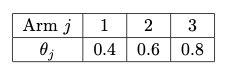Therefore, we have that

We design three algorithms as follows to maximize the aggregated reward.

#### $\epsilon$ - Greedy Algorithm

Main idea:

An $\epsilon$ - greedy policy is that simply explores (choose one arm randomly) with probability $\epsilon$ , or otherwise greedily exploits the information we already have and thereby choose the arm with current hightest reward.
Let $\hat{\theta}(j)$ be the average expectation reward for arm $j$ ($\ j\in\{1,2,3\}\$). $I(t)\in\{1,2,3\}$ denotes the arm we choose; $count$$(j)$ is the number of how many times arm $j$ has been chosen.
Therefore, for each time slots (we have N in total), we decide the arm $I(t)$ by explorarion or exploitation. After that, $count$($I(t)$) increases by 1. Suppose that arm $I(t)$ has been picked for $n$ times and results in reward $x_1,x_2,\dots,x_n$, $count(I(t)) = n+1$.

Pseudocode: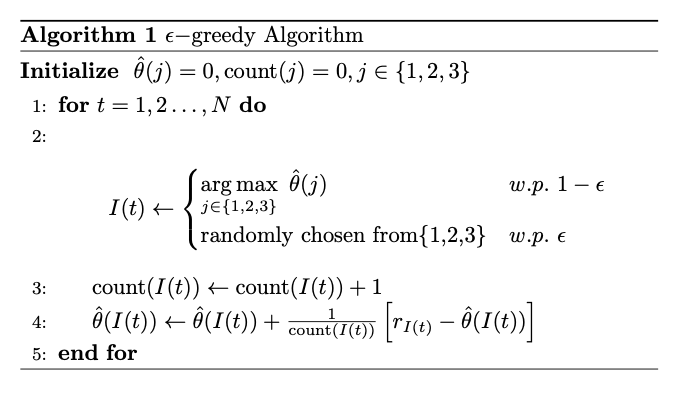Code and Results
We compute the expectation, and plot the actual reward and average reward against time slot ($t=1,2,\dots,N$). Due to the large scale of $N$, to see more clearly, we set the $x$ axis to be the $\lg N$.
To see the exact performance of each earm, we also plot the the reward of each arm. If the arm is not chosen, we set the reward to be -1 as a default assignment. Each time when a arm is chosen, we alternate the default reward ($-1$) with the actual reward ( $0$ or $1$ ).
To reduce the experiment error, we repeat the experiment for $100$ times and take the average values as outputs.
Here are the code and results.

epsilon:0.1
mean= 7787.06

epsilon:0.5
mean= 7002.36

epsilon:0.9
mean= 6199.36


According to the simulation, we observe that:

• $\epsilon$ denotes the probability of exploration. Therefore, when $\epsilon = 0.1$, the average reward almost converges to 0.8 (but still not reach 0.8, because we always has $\epsilon$ probability to explore), the actual reward almost oscillates near 0.8. We also see that as time goes by, we are more likely to choose arm 3 and only choose arm 1 or 2 in the exploration phase.
• Compared to $\epsilon = 0.1$, when $\epsilon$ is higher, we get worse aggregated expectation. Because when it comes to large time slot, we already know that the third arm deliver the best performance. If $\epsilon$ is still high and if we are still exploring, it would drag down the aggregated reward. Additionally, if $\epsilon$ is too high, we only have small probability to exploit arm3 as shown in the figure.

However, is it always the case that — when $\epsilon$ increases, we get worse performance? We set $\epsilon = 0.005, 0.01, 0.1, 0.3, 0.5, 0.75$, set $N=1500$ and do the simulation again.
Here are the code the result.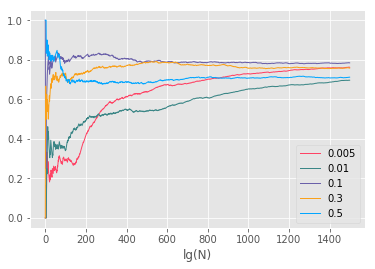$\ \ \$We notice that if $\epsilon$ is too small, we do too little exploration, therefore, we may not get an accurate understanding of the arm performance. Therefore, the total reward is decreased.

#### Improvement of $\epsilon$-Greedy Algorithm

$\ \ \$We design an improved $\epsilon$-Greedy algorithm to get better performance. The motivation is that — when $t$ is small, we set large $\epsilon$ to explore and obtain information quickly; when $t$ is large, we set small $\epsilon$ and exploit the information we already have. Shown as the follows, we set $\epsilon = t^{-\frac{1}{2}}$. We remain the $N=10000$ and repeat the experiments for 100 times just as previous experiments.

mean= 7956.26


As a result, we discovery that the new aggregated reward is much closer to 8000; the reward and average reward converge to 0.8 more quickly.

#### Upper Confidence Bound (UCB) Algorithm

Main idea:

$\ \ \$First, we pull each arm once for initialization.

$\ \ \$For $t = 4,\dots, N$, we choose the arm with highest Upper Confidence Bound (UCB).

where $\hat{\theta}(j)$ is the average reward observed from arm $j$ , $c$ is a constanst, $t$ is the number of attempts so far, $count(j)$ is the number of times arm $j$ has been pulled. In this formula, the first part is the exploitation term and the second part is the exploration term.

Pseudocode: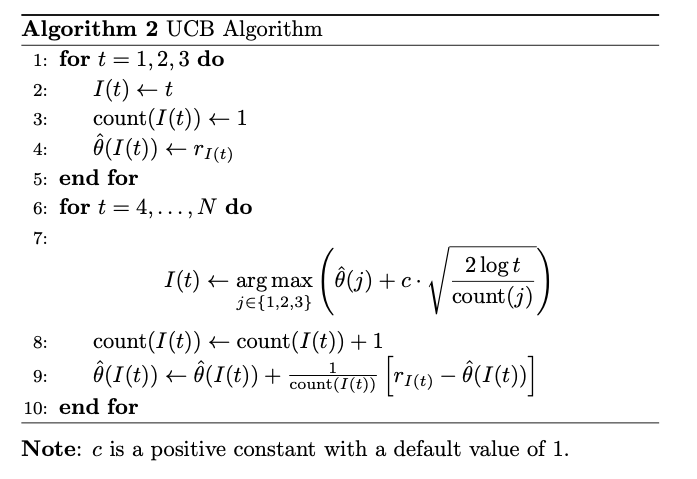Code and Results：

c:1
mean= 7892.49

c:5
mean= 7148.43

c:10
mean= 6676.07


According to the simulation:

• Each time the best arm is selected, $\hat{\theta}(j)$ would increase, however, $count(j)$ also increases and cause the second term to decrease. On the other hand, each time an action other than arm $j$ is selected, $t$ increases and the whole term increase, which cause the arm more likely to be choosen next time.
• The first term indicates the exploitation phase, and the second term indicates the exploration phase. Very silimar to the $\epsilon$ - Greedy Algorithm, if we set $c$ to be larger, then we do more exploration even $t$ is relatively large, and it would be very difficlut for the figure to converge to 0.8.

#### Improvement of UCB Algorithm

$\ \ \$We design an improved UCB algorithm to get better performance. The motivation is that — when $t$ is small, we set large $c$ to explore and obtain information quickly; when $t$ is large, we set small $c$ and exploit the information we already have. Shown as the follows, we set $c = \frac{1}{\log(t)}$. We remain the $N=10000$ and repeat the experiments for 100 times just as previous experiments.

mean= 7965.27


On average, we get better performance.
However, we also notice that, for some cases, the total reward is relatively small, the UCB algorithm is quite unstable and do not have a excellent lower bound.

#### Thompson Sampling Algorithm

Main idea:
We notice that in the UCB algorithm, we actually do not use the information of probability distribution.
The next algorithm, however, we take full advantage of the information that each arm generates a Bernoulli distributed reward.
In Thompson Sampling algorithm, we define a prior probability distribution for each arm, $\hat{\theta}(j)\sim\text{Beta}(\alpha_j,\beta_j)$. In the experiment, for arm $j$, if we observe there are $k$ success out of $m$ attempts, then we update the probability, i.e., $\hat{\theta}(j)\sim\text{Beta}(\alpha_j+k, \beta_j+n-k)$.
The proof is shown as follows.

In the simulation, we first pull each arm once as initialization. For $t = 4,\dots, N$, we always pull the arm with highest $\hat{\theta}$.

Pseudocode: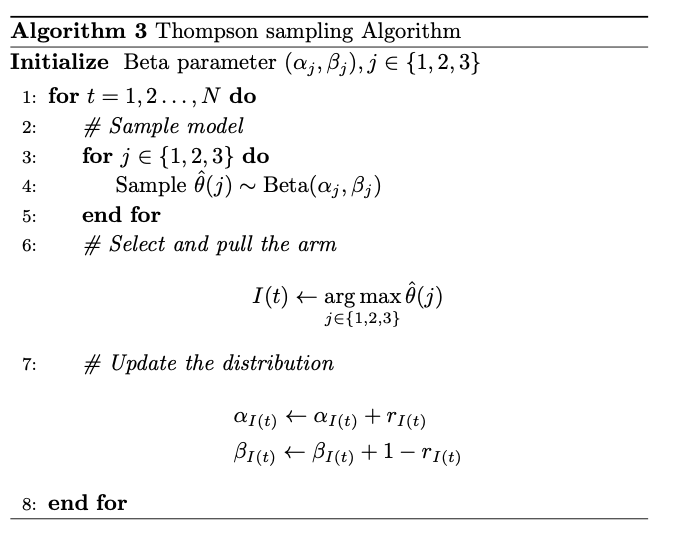Code and Results:

alpha1 = 1
beta1 = 1
alpha2 = 1
beta2 = 1
alpha3 = 1
beta3 = 1
mean= 7988.33

alpha1 = 2
beta1 = 4
alpha2 = 3
beta2 = 6
alpha3 = 1
beta3 = 2
mean= 7988.64


According to the simulation:

• Different from $\epsilon$ - Greedy and UCB algorithm, as $t\rightarrow \infty$, the probability of chosen arm 1 and arm 2 almost converge to 0.
• We notice that the prior distribution only makes small difference to the final reward. However, if the prior probability is more closer to the true probability, it would take less time to find out which arm is the best.

### Summary

The overall reults is shown as follows: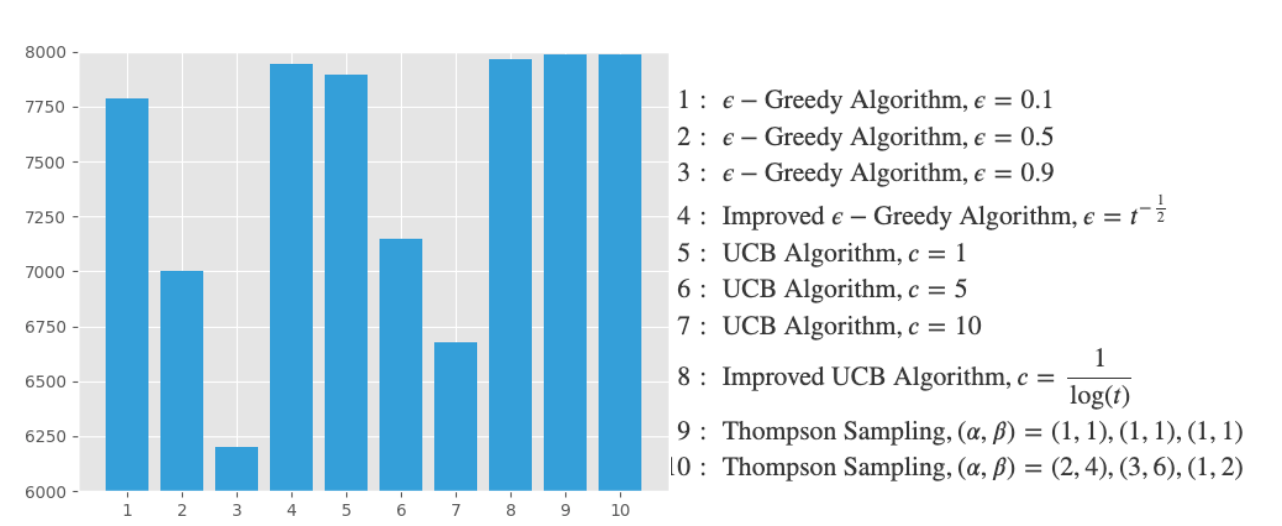### Dependent Cases

In the previous situation, we assume the reward distribution of three arms are independent. Here, we provide a method to analyze the dependent multi-armed bandit problems.

#### Models

To generalize the problem, we assume that there is a machine with $N$ arms that are grouped into $K$ clusters. Every arm in a single cluster are dependent, but arms in different clusters are independent of each other. Each arm $i$ has a fixed but unknown probability $\theta(i)$ of generating a success reward (i.e. $r_i = 1$). Let $[i]$ denotes the whole arm cluster which contains the $i$th arm, and define $C_{[i]}$ be the set of all arms in that cluster including the $i$th arm.
Define $s_i(t)$ be the number of times arm $j$ successfully generates a reward in $t$ time slot, $f_i(t)$ be the number of times that arm $i$ fails to generate a reward, $\varphi(h_{[i]})$ be the prior probability distribution and function $h_{[i]}$ abstract out the dependence of arms on each other in one cluster.
Therefore, we have that

In each timeslot, we solve the problem in two steps:

1. Selection step: Apply a specific policy to choose the arm to pull.
2. Update step: Use the result of the arm pull to update the information.


We should notice the difference between independent and the dependent cases. In a independent situation, once arm $j$ is pulled, only the information of arm $i$ is updated. However, in the dependent cases, once an arm $i$ is chosen, the state of all arms in $C_{[i]}$ is changed.

#### Method

In each time slot, for each cluster $i$, we computer the reward estimate $\hat{\theta}(i)$ and the corresponding estimate variance $\hat{\sigma}(i)$ of each cluster. After that, we use a specfic policy (such as UCB, Thompson Sampling, etc.) to select a cluster. Finally, we use the policy to choose the “best” arm in that cluster. In this method, a good estimate reward should be accurate and converge quickly (i.e., $\hat{\sigma}(j)\to 0$ quickly).
We have two strategies shown as follows.

• Mean Strategy:
• For all arm $j$ in cluster $C_j$, according to the Binomial distribution, we set the cluster characteristics as follows:Here, the $s_j, f_j$ are posterior values.
• However, we notice that in the mean strategy, if there is one “bad” arm in a cluster, then the total estimate reward is dragged down. Therefore, it might be difficult to find the best rewarded arm if its siblings perform terribly. So, we design another algorithm shown as follows.
• Max Strategy:
• In this strategy, each cluster is represented by the arm that is currently best in it. That is:
• Compared to the mean strategy, this method would be closer to maximum success probability of cluster $j$. Because $\hat{\theta}(j)$ is not dragged down by its families.
• However, this method neglects all observations of other arms in that cluster and does not take full advantage of all current information.

### Reference

1. Slivkins, A. (2019). Introduction to multi-armed bandits. Foundations and Trends in Machine Learning. https://doi.org/10.1561/2200000068
2. Pandey, Sandeep & Chakrabarti, Deepayan & Agarwal, Deepak. (2007). Multi-armed bandit problems with dependent arms.. 721-728.
3. Connor Lee, Hoang Le, R. M. (2016). Multi-armed Bandits. Retrieved from http://www.yisongyue.com/courses/cs159/